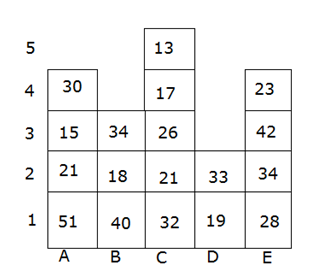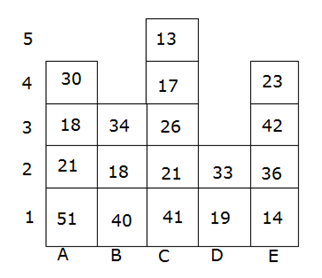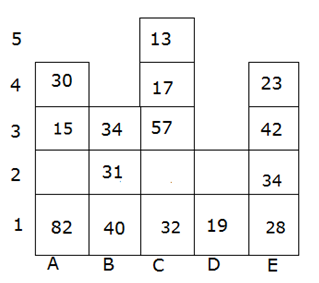# IBPS Clerk Mains Reasoning (Day-16)

Dear Aspirants, Our IBPS Guide team is providing new series of Reasoning Questions for IBPS Clerk Mains 2021 so the aspirants can practice it on a daily basis. These questions are framed by our skilled experts after understanding your needs thoroughly. Aspirants can practice these new series questions daily to familiarize with the exact exam pattern and make your preparation effective.

Start Quiz

Puzzle

Direction (1-4): Read the given information carefully and answer the following questions.

There are 5 buildings A, B, C, D, and E such that building A is to the west of building B, building B is to the west of building C and so on. Building A has four floors, building B has three floors, building C has five floors, building D has two floors and building E has 4 floors. The bottommost floor is numbered 1, the floor above it is numbered 2 and so on.

The number of persons on each building from the bottommost floor to the topmost floor is given as follows.

Building A: 51, 21, 15, 30

Building B: 40, 18, 34

Building C: 32, 21, 26, 17, 13

Building D: 19, 33

Building E: 28, 34, 42, 23

A dice throwing game is being played and 3 dices are thrown. As per the given conditions, the movements of persons take place.

I)If a prime number appears on the third dice and an even number appears on at least one of the other two dices, then the persons on the third floor of the building that is immediately next in the east direction to the building with the least number of floors moves to the third floor of the building that is immediately next in the west direction to the building having a maximum number of floors.

II)If a prime number appears on more than one of the dices, and an even number appears on the other dice then half the number of persons on the first floor of building E is moved to the second floor of the same building and then one – fourth of the total persons in the second-floor of that building moves to the third floor of building A and then one – third of the persons on the third floor of building A moves to the first floor of building C.

III) If an odd prime number appears on either of the first or third dice and two non-prime numbers appear on the other two dices then the persons on the second floors of all the first four buildings from west to the east together are equally distributed to the first three buildings from west to east. The persons move in such a way that the persons distributed to building A move to the first floor of building A, the persons distributed to building B move to the second floor of building B, and the remaining to the third floor of building C.

(Note: Take 1 as a non-prime number)

1) What will be the total number of persons in the buildings B and C together if 4, 2, and 5 appear on throwing the three dices respectively?

A.263

B.265

C.273

D.241

E. data insufficient

2) What will be the total number of persons in the buildings A, C, and E together if 3, 2, and 6 appear on throwing the three dices respectively?

A.340

B.353

C.343

D.334

E. data insufficient

3) On throwing the three dices, 5, 1, and 4 appear respectively. Then the number of persons on the even-numbered floors of all the buildings together is _____?

A.125

B.146

C.135

D.153

4) On throwing the three dices, different numbers appear on each dice and then a certain number of persons have moved from one building to another building accordingly. If the number of persons on the buildings B and E is 134, 85 respectively, then which of the following represents the outcomes of three dices up on throwing?

A.8, 2, and 3

B.5, 1, and 3

C.1, 4, and 3

D.6, 2, and 5

E.None of these

Critical reasoning

Directions (5): the question below is given two statements I and II. These statements may be either independent causes or maybe the effects of independent causes or a common cause. One of the statements may be the effect of the other statements. Read both the statements and decide which of the answer choice correctly depicts the relationship between these two statements.

10)  Statements:

I). The Art of Living (AoL), headed by spiritual leader Ravi Shankar, today alleged that the expert committee appointed by the National Green Tribunal had tried to “distort and mislead” it on the damage caused to the Yamuna floodplains.

II). Earlier, the expert’s committee had told the NGT that whopping Rs 42.02 crore would be required to restore the Yamuna floodplains which were ravaged due to the AoL’s cultural event.

A. Statement I is the cause and statement II is its effect

B. Statement II is the cause and statement I is its effect

C.Both the statements I and II are independent causes

D. Both the statements I and II are effects of independent causes

E. Both the statements I and II are effects of some common cause

Input output

Directions (6-9): A machine rearrangement given an input line having both words and numbers in a particular set of rules in step by step. The following is an illustration of input and its rearrangement.

Input: strong 4348 change 7321 brand 1025 1302 cause 6341 great 2712 surface

Step I: brand strong 4348 change 7321 1025 cause 1302 6341 great 2712 surface

Step II: brand 8 strong 4348 change 7321 cause 6 6341 great 2712 surface

Step III: brand 8 change 4348 strong 7321 cause 6 great 6341 2712 surface

Step IV: brand 8 change 13 4348 strong cause 6 great 12 6341 surface

Step V: brand 8 change 13 strong 4348 cause 6 great 12 surface 6341

Step VI: brand 8 change 13 strong 19 cause 6 great 12 surface 14

As per rules followed in the given steps, find appropriate step for given Input.

Input: 7234 science 3416 2103 global contest 5943 last occur 3121 glitch 2643

6) Which of the following element is sixth from the left end in step IV?

A.glitch

B.science

C.3416

D.7234

E.None of these

7) Which of the following step represents the following output ‘contest 6 global 14 science 7234 glitch 7 occur 15 last 5943’?

A.There is no such step

B.Step IV

C.Step II

D.Step III

E. Step I

8) How many elements are there between ‘science’ and ‘last’ in step II?

A.Seven

B.Three

C.Five

D.Six

E.None of these

9) What is the sum of the third element from the right end in the last step and the fourth element from the left end in step III?

A.7250

B.7249

C.21

D.35

E.None of these

Misc

10) Four of the five among the following five are similar in such a way to form a group, which one among the following doesn’t belong to the group?

A.QS

B.MC

C.SG

D.WK

E.TL

Directions (1-4) :

From the given information, the diagrammatic representation of the buildings, its floors, and the number of persons on each floor are as follows.The dices show 4, 2, and 5 respectively.

Here the third dice shows a prime number and the other two dices shows even numbers. So condition I may apply.

But 2 is an even prime number here. So condition II may also apply.

So, the data is not sufficient enough to answer the question.

On throwing the dices, they show 3, 2, and 6 respectively.

Here 2 and 3 are prime numbers and 6 is an even number. So condition II applies here.

As per condition II, half the number of persons on the first floor of the building E i.e. 14 persons moves to the second floor of the same building. So, the number of persons on the second floor of building E will be 34 + 14 = 48.

Now, one – fourth of the persons on the second floor of the building E i.e. 12 persons have to be moved to the third floor of building A. Then the number of persons on the third floor of building A will be 15 + 12 = 27.

Now, one – third of the number of persons on the third floor of building A i.e. 9 persons have to be moved to the first floor of building C. Then the number of persons on the first floor of building C will be 32 + 9 = 41.

After following all the steps, the number of persons on different floors of different buildings is as follows.The total number of persons in buildings A, C, and E =120 + 118 + 115= 353

The first dice shows a prime number and the other two dices are non-prime numbers. So, condition iii applies here.

As per condition III, the persons on the second floors of the first four buildings together i.e. 93 has to be equally distributed to the buildings A, B, and C. So 31 persons will be added to each building.

It is also given that they move in such a way that persons distributed to building A move to the first floor of building A, persons distributed to building B move to the second floor of building B, and the remaining to the third floor of building C.The number of persons on the even-numbered floors of all the buildings together = 31 + 34 +30 +17 + 23 = 135

If we can observe clearly, the persons from the third floor of building E have moved to build B, which means the condition I is applied here.

So, the number on the third dice must be a prime number and any of the other two must be an even number. Option b can be eliminated for the same reason.

In option a and option d, there is an even prime number i.e.2. None of them could be the outcomes because if they are the outcomes, then it would make a clash between condition I and condition II. The data is insufficient in their case.

So, option c is correct.

Statement II states that the expert’s committee had told the NGT that a certain amount of money would be needed to restore the damage done by Aol’s event. This has led the Aol to allege that there has been a distortion of data regarding the damage. Hence statement II is the cause and the effect is statement I. Thus, option B is the correct answer.

Directions (6-9):

Logic:

In the given input there are twelve elements, in which the first six elements are arranged in one logic, the same logic which is used for the second six elements

The first six elements are arranged in which words are arranged in ascending order and the numbers are arranged in ascending order with respect to the sum of the digits in a number.

For the second six elements, the same logic is applied as in the first six elements.

Input: 7234 science 3416 2103 global contest 5943 last occur 3121 glitch 2643

Step I: contest 7234 science 3416 2103 global glitch 5943 last occur 3121 2643

Step II: contest 6 7234 science 3416 global glitch 7 5943 last occur 2643

Step III: contest 6 global 7234 science 3416 glitch 7 last 5943 occur 2643

Step IV: contest 6 global 14 7234 science glitch 7 last 15 5943 occur

Step V: contest 6 global 14 science 7234 glitch 7 last 15 occur 5943

Step VI: contest 6 global 14 science 16 glitch 7 last 15 occur 21